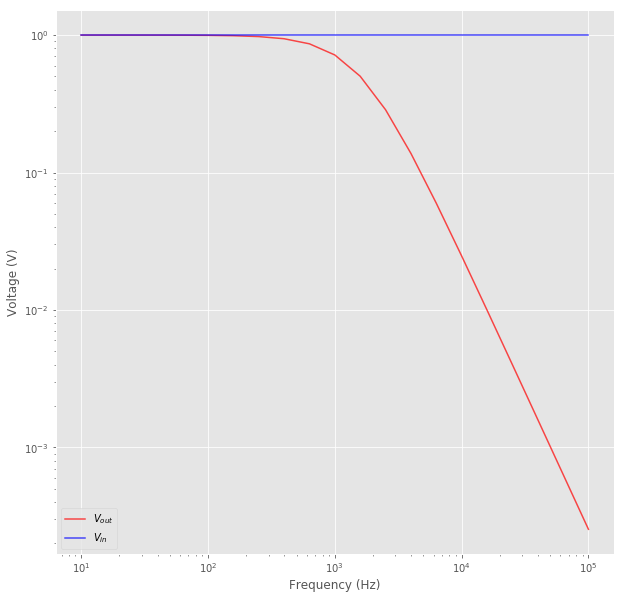## How to Simulate Circuit Netlists Directly in a Jupyter Notebok

This blog post is viewable as a Jupyter notebook! [Link]

The Jupyter Notebook is one of the coolest open source data processing application out there. If you aren't familiar, it essentially is a web-based IDE that allows one to combine code of numerous languages (particularly Python, Julia, and R) with markdown and inline plots and graphs, meaning one can annotate what they are doing with $\LaTeX$ style equations, code in the language of their choice, and have the code generate a plot that is created in the same file, or "notebook."

As an electrical engineer, I have been thinking to myself, "boy wouldn't it be nice to be able to integrate ngspice into Jupyter notebooks so I can modify my netlists and see the resulting plots on the fly?" I figured out how to do exactly that. And the solution is quite elegant too.

### Pre-requisites:

You need to have the following installed and be able to use them for this example:

• Python3
• Matplotlib & Numpy Python packages
• Jupyter
• Ngspice (I use version 30, but it really doesn't matter, you can likely use any SPICE simulator)

Caveat: There is no way to have the ngspice generated plots displayed in-line, below the code in the Jupyter notebook, as one would expect with most Jupyter plots. Ngspice generates a postscript plot, and while I am sure there is a funny way to convert it and display it, I figured I am already in a python environment, so why not just have Ngspice write the data into a whitespace seperated file and then have python read and plot it using matplotlib? You are welcome to use your preferred plotting system instead, of course.

We will start with importing and configuration:

input :

%matplotlib inline
import numpy as np
import matplotlib.pyplot as plt

plt.style.use('ggplot') # I like this style


The following cell is the SPICE netlist. It's just a simple RC low-pass filter.

To tell Jupyter that this is not ordinary python code, we use the script magic in the first line (see documentation here: https://ipython.readthedocs.io/en/stable/interactive/magics.html#cellmagic-script). Basically, we are telling jupyter to run ngspice as a subprocess, with everything below the first line as the input file. Jupyter will then will print Ngspice's stdout below the cell.

For example, here I am telling Ngspice to print Vout. The result is printed after we run the cell. We can then use the wrdata Ngspice command to print Vout and Vin to example.data.

input :

%%script ngspice
basic RC circuit
*#destroy all
*#run
*#print Vout
*#wrdata example.data Vout Vin
*#exit

R1 Vin Vout 1k
C1 Vout Gnd 100n
Vin Vin Gnd DC 0 AC 1

.AC dec 5 10 100k

.end


output :

Circuit: basic rc circuit

Doing analysis at TEMP = 27.000000 and TNOM = 27.000000

No. of Data Rows : 21
basic rc circuit
AC Analysis  Thu Mar 28 11:41:24  2019
--------------------------------------------------------------------------------
Index   frequency       vout
--------------------------------------------------------------------------------
0   1.000000e+01    9.999605e-01,   -6.28294e-03
1   1.584893e+01    9.999008e-01,   -9.95719e-03
2   2.511886e+01    9.997510e-01,   -1.57787e-02
3   3.981072e+01    9.993747e-01,   -2.49982e-02
4   6.309573e+01    9.984308e-01,   -3.95820e-02
5   1.000000e+02    9.960677e-01,   -6.25848e-02
6   1.584893e+02    9.901808e-01,   -9.86040e-02
7   2.511886e+02    9.756962e-01,   -1.53991e-01
8   3.981072e+02    9.411153e-01,   -2.35409e-01
9   6.309573e+02    8.641799e-01,   -3.42597e-01
10  1.000000e+03    7.169568e-01,   -4.50477e-01
11  1.584893e+03    5.020955e-01,   -4.99996e-01
12  2.511886e+03    2.864575e-01,   -4.52106e-01
13  3.981072e+03    1.377997e-01,   -3.44690e-01
14  6.309573e+03    5.982063e-02,   -2.37154e-01
15  1.000000e+04    2.470452e-02,   -1.55223e-01
16  1.584893e+04    9.983497e-03,   -9.94174e-02
17  2.511886e+04    3.998529e-03,   -6.31074e-02
18  3.981072e+04    1.595683e-03,   -3.99141e-02
19  6.309573e+04    6.358637e-04,   -2.52083e-02
20  1.000000e+05    2.532388e-04,   -1.59115e-02
ngspice-30 done


Now all we need to do is process example.data with python3, though you can use any Jupyter-compatible language.

input :

data = np.genfromtxt("example.data")

fig, ax = plt.subplots()

ax.plot(data[:, 0], data[:, 1], 'r-', label='$V_{out}$', alpha=0.7)
ax.plot(data[:, 3], data[:, 4], 'b-', label='$V_{in}$', alpha=0.7)
ax.set(xlabel="Frequency (Hz)", ylabel="Voltage (V)")
ax.set_xscale("log")
ax.set_yscale("log")
ax.legend()


output :

<matplotlib.legend.Legend at 0x7fdc818c9940>And of course, we can do whatever other data processing we want with python (and there is a lot you can do there). That just about wraps it up. Happy circuit simulating!